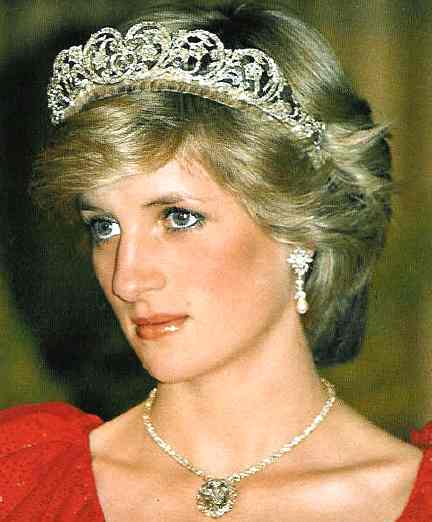## people with a 25 (Knight of Wands) life lesson

### July 5, 1999people with a 25 life lessonborn July 1st, 1961

7 + 1 +1+9+6+1 = 25 = her life lesson = what she was here to learn = Adventure.  Excitement.  Thrilling.  Movement.  Exploring.  Activist.  Advocate.  A cause.  Rooting.  Encouragement.  Free spirited.  Perky.

——-

Joan Baez
born January 9th, 1941                 1 + 9 +1+9+4+1 = 25

Chuck D.
born August 1st, 1960                   8 + 1 +1+9+6+0 = 25

Martin Sheen
born August 3rd, 1940                  8 + 3 +1+9+4+0 = 25

Vicente Fox
born July 2nd, 1942                      7 + 2 +1+9+4+2 = 25

Josephine Baker
born June 3rd, 1906                     6 + 3 +1+9+0+6 = 25

Dr. Phil
born September 1st, 1950             9 + 1 +1+9+5+0 = 25

Mel Gibson
born January 3rd, 1956                 1 + 3 +1+9+5+6 = 25

Marilyn Monroe
born June 1st, 1926                      6 + 1 +1+9+2+6 = 25

Farrah Fawcett
born February 2nd, 1947              2 + 2 +1+9+4+7 = 25

Tchaikovsky
born May 7th, 1840                      5 + 7 +1+8+4+0 = 25

Stephen Hawking
born January 8th, 1942                1 + 8 +1+9+4+2 = 25

Lupita Nyong’o

born March 1st, 1983                    3 + 1 +1+9+8+3 = 25

Willie Mays
born May 6th, 1931                     5 + 6 +1+9+3+1 = 25

J. Edgar Hoover
born January 1st, 1895                 1 + 1 +1+8+9+5 = 25

Dan Akroyd
born July 1st, 1952                      7 + 1 +1+9+5+2 = 25

Dom Deluise
born August 1st, 1933                  8 + 1 +1+9+3+3 = 25

Oliver Wendell Holmes
born March 8th, 1841                   3 + 8 +1+8+4+1 = 25

Carl Lewis
born July 1st, 1961                      7 + 1 +1+9+6+1 = 25

Samuel J. Tilden
born February 9th, 1814               2 + 9 +1+8+1+4 = 25

Andy Griffith
born June 1st, 1926                     6 + 1 +1+9+2+6 = 25

Jennifer Jason Leigh
born February 5th, 1962              2 + 5 +1+9+6+2 = 25

Lon Chaney
born April 1st, 1883                    4 + 1 +1+8+8+3 = 25

George Melville
born January 10th, 1841             1 + 10 +1+8+4+1 = 25

Albert Spalding
born September 2nd, 1850            9 + 2 +1+8+5+0 = 25

Rick James
born February 1st, 1948                2 + 1 +1+9+4+8 = 25

Dyan Cannon
born January 4th, 1937                 1 + 4 +1+9+3+7 = 25

Charles Osgood
born January 8th, 1933                 1 + 8 +1+9+3+3 = 25

Olivia de Havilland
born July 1st, 1916                       7 + 1 +1+9+1+6 = 25

Franco Harris
born March 7th, 1950                   3 + 7 +1+9+5+0 = 25

Jack Paar
born May 1st, 1918                      5 + 1 +1+9+1+8 = 25

Peter O’Toole
born August 2nd, 1932                 8 + 2 +1+9+3+2 = 25

Fabian
born February 6th, 1943              2 + 6 +1+9+4+3 = 25

Jamie Farr
born July 1st, 1934                     7 + 1 +1+9+3+4 = 25

Tony Orlando
born April 3rd, 1944                    4 + 3 +1+9+4+4 = 25

Sydney Pollack
born July 1st, 1934                     7 + 1 +1+9+3+4 = 25

Alan Thicke
born March 1st, 1947                  3 + 1 +1+9+4+7 = 25

Robert Wagner
born February 10th, 1930             2 + 10 +1+9+3+0 = 25

Picabo Street
born April 3rd, 1971                    4 + 3 +1+9+7+1 = 25

Don Sutton
born April 2nd, 1945                    4 + 2 +1+9+4+5 = 25

Tyrone Power
born May 5th, 1914                     5 + 5 +1+9+1+4 = 25

Rocky Marciano
born September 1st, 1923              9 + 1 +1+9+2+3 = 25